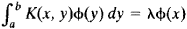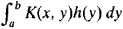# Eigenfunction

Also found in: Dictionary, Medical, Wikipedia.
Related to Eigenfunction: eigenfunction expansion

## Eigenfunction

One of the solutions of an eigenvalue equation. A parameter-dependent equation that possesses nonvanishing solutions only for particular values (eigenvalues) of the parameter is an eigenvalue equation, the associated solutions being the eigenfunctions (sometimes eigenvectors). In older usage the terms characteristic equation and characteristic values (functions) are common. Eigenvalue equations appear in many contexts, including the solution of systems of linear algebraic equations (matrix equations), differential or partial differential equations, and integral equations. The importance of eigenfunctions and eigenvalues in applied mathematics results from the widespread applicability of linear equations as exact or approximate descriptions of physical systems. However, the most fundamental application of these concepts is in quantum mechanics where they enter into the definition and physical interpretation of the theory. Only linear eigenvalue equations will be discussed. See Eigenvalue (quantum mechanics), Energy level (quantum mechanics), Quantum mechanics

McGraw-Hill Concise Encyclopedia of Physics. © 2002 by The McGraw-Hill Companies, Inc.
The following article is from The Great Soviet Encyclopedia (1979). It might be outdated or ideologically biased.

## Eigenfunction

a concept of mathematical analysis. The solution of many problems in mathematical physics—for example, in the theory of vibrations and the theory of heat conduction—requires the finding of nontrivial solutions of homogeneous linear differential equations L(y) = Xy where the solutions satisfy particular boundary conditions. Such solutions are called ei-genfunctions of the problem, and the corresponding values of λ are called eigenvalues.

If a differential equation with appropriate boundary conditions is self-adjoint, then its eigenvalues are real, and the eigenfunctions corresponding to distinct eigenvalues are orthogonal. If the differential equation is considered on a finite interval and its coefficients have no singularities on the interval, then the set of eigen-functions is countable—that is, the problem has a discrete spectrum. If we know the eigenfunctions and the corresponding eigenvalues, then, under certain conditions, we can obtain a solution of the problem in the form of a series of eigenfunctions. Suppose, however, the equation is considered on an infinite interval or its coefficients have singularities (for example, the coefficient of the derivative of highest order takes on the value 0). A continuum of eigenfunctions may then exist, and expansion in the form of an integral with respect to the eigenfunctions is obtained instead of a series expansion. Such an integral is analogous to a Fourier integral representation. In this case, the problem is said to have a continuous spectrum. Many special functions, such as orthogonal polynomials, serve as the eigenfunctions of some equations.

In the theory of integral equations, a function that for some value of X satisfies the equationis called an eigenfunction of the kernel K(x, y). Any symmetric continuous kernel has an eigenfunction. In this case, any function representable in the formcan be expanded in a series of eigenfunctions. If the kernel has singularities or is defined in an infinite domain, then a continuous spectrum may also arise.

Eigenfunctions can be defined most generally as the eigenvectors of linear operators in linear function spaces. In quantum mechanics, the eigenfunctions of an operator corresponding to some physical quantity correspond to those states of a system in which the given physical quantity has a definite value.

Eigenfunctions are sometimes also called proper functions or characteristic functions.

The Great Soviet Encyclopedia, 3rd Edition (1970-1979). © 2010 The Gale Group, Inc. All rights reserved.

## eigenfunction

[′ī·gən‚fəŋk·shən]
(mathematics)
Also known as characteristic function.
An eigenvector for a linear operator on a vector space whose vectors are functions. Also known as proper function.
A solution to the Sturm-Liouville partial differential equation.
McGraw-Hill Dictionary of Scientific & Technical Terms, 6E, Copyright © 2003 by The McGraw-Hill Companies, Inc.
References in periodicals archive ?
To find the first eigenvalues and eigenfunctions of the problem (3.3) with sparse matrices A and B, one can use various numerical methods .
The second eigenfunction accounted for 15.21 percent of total genetic variation and had positive and constant behavior during lactation period, suggesting that all of the variation can be explained by genes that operate in similar way during lactation.
This space is then enriched with functions that are adaptively selected eigenfunctions of a specially constructed generalized eigenvalue problem (cf.
as the decomposition for the solution of (1), where [mathematical expression not reproducible], are the eigenvalue and eigenfunction pairs for the -[DELTA] + V operator.
Once the eigenvalue [[lambda].sup.(c)] and eigenfunction [f.sup.(c).sub.n](x) of conditional covariance function are obtained, the conditional log hydraulic conductivity random field can be generated using
Clearly, r and [psi] are the eigenvalue and eigenfunction of [T.sub.k] if and only if [mu] = r - k and [psi] are the corresponding eigenvalue and eigenfunction of the problem
In Section 2, the theory utilized to describe the plasma structure in both the eigenfunction solution and the FDTD simulation is briefly outlined.
In Section 3, the eigenfunctions of scalar bosons in the presence of the Aharonov-Bohm and Coulomb potentials in the gravitational field of topological defects such as cosmic string and global monopole have been discussed in Sections 3 and 4.
In the last case, the eigenfunctions of the two states are different and orthogonal while those of two coalescing eigenstates of H are biorthogonal and differ only by a phase from one another at the EP.
In addition, based on the homogeneous boundary conditions for the depth given in (14), the eigenfunction of [mathematical expression not reproducible] is sin(Mz/H).
We then develop the wave function, [psi], of this system with the eigenfunction [[psi].sub.r] of [H.sub.0], such as [psi] = [[summation].sub.r][b.sup.r][[psi].sub.r], where [[absolute value of ([b.sub.r])].sup.2] corresponds to the probability of this system being in the state r.
where [[phi].sub.k]([[xi].sup.[alpha]]) and [[??].sub.k]([[xi].sup.[alpha]]) denote, respectively, the exact and the approximate kth eigenfunction. Note that (see (47))

Site: Follow: Share:
Open / Close